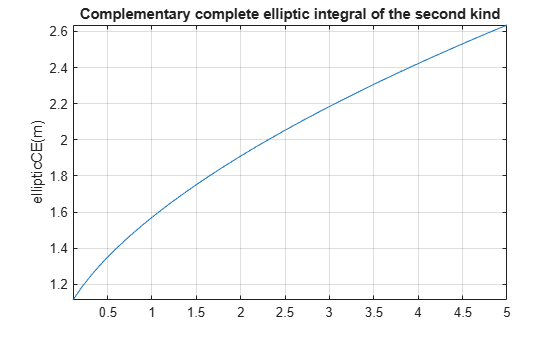# ellipticCE

Complementary complete elliptic integral of the second kind

## Syntax

``ellipticCE(m)``

## Description

example

````ellipticCE(m)` returns the complementary complete elliptic integral of the second kind.```

## Examples

### Find Complementary Complete Elliptic Integral of the Second Kind

Compute the complementary complete elliptic integrals of the second kind for these numbers. Because these numbers are not symbolic objects, you get floating-point results.

```s = [ellipticCE(0), ellipticCE(pi/4),... ellipticCE(1), ellipticCE(pi/2)]```
```s = 1.0000 1.4828 1.5708 1.7753```

Compute the complementary complete elliptic integrals of the second kind for the same numbers converted to symbolic objects. For most symbolic (exact) numbers, `ellipticCE` returns unresolved symbolic calls.

```s = [ellipticCE(sym(0)), ellipticCE(sym(pi/4)),... ellipticCE(sym(1)), ellipticCE(sym(pi/2))]```
```s = [ 1, ellipticCE(pi/4), pi/2, ellipticCE(pi/2)]```

Use `vpa` to approximate this result with floating-point numbers:

`vpa(s, 10)`
```ans = [ 1.0, 1.482786927, 1.570796327, 1.775344699]```

### Find Elliptic Integral for Matrix Input

Call `ellipticCE` for this symbolic matrix. When the input argument is a matrix, `ellipticCE` computes the complementary complete elliptic integral of the second kind for each element.

`ellipticCE(sym([pi/6 pi/4; pi/3 pi/2]))`
```ans = [ ellipticCE(pi/6), ellipticCE(pi/4)] [ ellipticCE(pi/3), ellipticCE(pi/2)]```

### Differentiate Complementary Complete Elliptic Integral of the Second Kind

Differentiate these expressions involving the complementary complete elliptic integral of the second kind:

```syms m diff(ellipticCE(m)) diff(ellipticCE(m^2), m, 2)```
```ans = ellipticCE(m)/(2*m - 2) - ellipticCK(m)/(2*m - 2) ans = (2*ellipticCE(m^2))/(2*m^2 - 2) -... (2*ellipticCK(m^2))/(2*m^2 - 2) +... 2*m*(((2*m*ellipticCK(m^2))/(2*m^2 - 2) -... ellipticCE(m^2)/(m*(m^2 - 1)))/(2*m^2 - 2) +... (2*m*(ellipticCE(m^2)/(2*m^2 - 2) -... ellipticCK(m^2)/(2*m^2 - 2)))/(2*m^2 - 2) -... (4*m*ellipticCE(m^2))/(2*m^2 - 2)^2 +... (4*m*ellipticCK(m^2))/(2*m^2 - 2)^2)```

Here, `ellipticCK` represents the complementary complete elliptic integral of the first kind.

### Plot Complementary Complete Elliptic Integral of Second Kind

Plot the complementary complete elliptic integral of the second kind.

```syms m fplot(ellipticCE(m)) title('Complementary complete elliptic integral of the second kind') ylabel('ellipticCE(m)') grid on```## Input Arguments

collapse all

Input, specified as a number, vector, matrix, or array, or a symbolic number, variable, array, function, or expression.

collapse all

### Complementary Complete Elliptic Integral of the Second Kind

The complementary complete elliptic integral of the second kind is defined as E'(m) = E(1–m), where E(m) is the complete elliptic integral of the second kind:

`$E\left(m\right)=E\left(\frac{\pi }{2}|m\right)=\underset{0}{\overset{\pi /2}{\int }}\sqrt{1-m{\mathrm{sin}}^{2}\theta }d\theta$`

Note that some definitions use the elliptical modulus k or the modular angle α instead of the parameter m. They are related as m = k2 = sin2α.

## Tips

• `ellipticCE` returns floating-point results for numeric arguments that are not symbolic objects.

• For most symbolic (exact) numbers, `ellipticCE` returns unresolved symbolic calls. You can approximate such results with floating-point numbers using `vpa`.

• If `m` is a vector or a matrix, then `ellipticCE(m)` returns the complementary complete elliptic integral of the second kind, evaluated for each element of `m`.

 Milne-Thomson, L. M. “Elliptic Integrals.” Handbook of Mathematical Functions with Formulas, Graphs, and Mathematical Tables. (M. Abramowitz and I. A. Stegun, eds.). New York: Dover, 1972.

## Support

#### Mathematical Modeling with Symbolic Math Toolbox

Get examples and videos